CATEGORIES:

# The direction of a vector. Let us find the angle between two vectors and .

Consider the inner product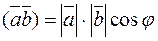.

We have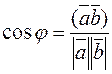. (*)

Writing the product and absolute values in coordinates, we obtain. (**)

Example 3. Find an angle between vectors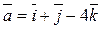and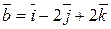. By using formula (**), we find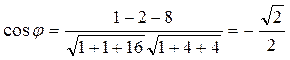,Let us determine a condition for vectors to be perpendicular. Suppose that vectors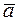andare perpendicular, i.e.,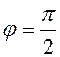; then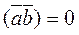, and. (7)This is the condition for vectors to be perpendicular.

z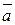0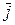y

x

Consider the angles between a vectorand the unit vectors. We denote these angles by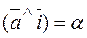;;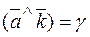.

Take the product ofand any unit vector, say,=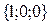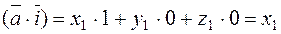.

By formula (*), the cosine of the angle a from it is.

Similarly the cosines of the other angles are,,. (8)

These cosines are called the directional cosines of the vector.

The sum of the squared directional cosines equals one: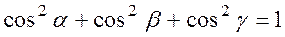.

To prove this, it sufficies to square the cosines by formula (8) and sum them: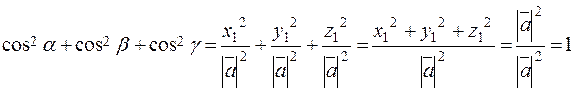.

Example 5. For what a are the vectorsandperpendicular?

We use the perpendicularity condition (7) and write the inner product of the given vectors in coordinates: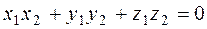;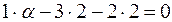, a=10.

Date: 2015-01-02; view: 1576

 <== previous page | next page ==> Decomposition of vectors. | Vector Product and Its Properties
doclecture.net - lectures - 2014-2023 year. Copyright infringement or personal data (0.013 sec.)